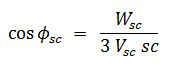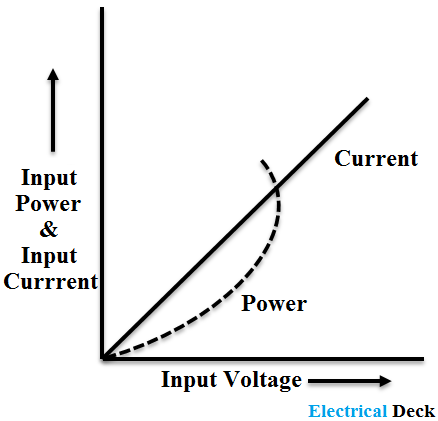# What is Blocked Rotor Test of Induction Motor ?

The blocked rotor test, it is quite opposite of the no-load test of the induction motor. Usually in transformer tests, if we want to find the behavior of a transformer on loading and on no-load, it can be done by using open circuit and short circuit tests. Here to know the behavior of a transformer when it is in the fully-loaded condition we have to perform the short-circuit test.

Similarly, the blocked rotor test is performed to know the behavior of an induction motor when it is fully loaded i.e., as same as the short-circuit test. The purpose of this is to determine the total resistance (R01) and reactance (X01) of the motor when referred to as the stator. This test is also used to know the amount of sound produced by the motor when it is fully loaded and is also used to draw the circle diagram.

The rotor, in this test, is prevented from rotating by a brake, and therefore it is called a blocked rotor test. The induction motor in this case acts like a short-circuited transformer.

## Process of Blocked Rotor Test :

A low voltage 3-phase supply (obtained from a 3-phase auto-transformer) is applied to the stator. So that full load current flows through the stator winding. The power input is measured by the two wattmeters. The short circuit current corresponding to the rated voltage can be calculated by direct proportion.

In the blocked rotor test, it voltage applied to the stator terminals must be low otherwise it can lead to damage to the stator winding. In this test, the low voltage is applied so that the there rotation of the rotor and motor draws a full load current through the stator winding.

## Calculation of Blocked Rotor Test :

#### When the rotor is blocked, slip s = 1 and the approximate equivalent circuit referred to the stator is shown below.Let,Wsc = Total power inputVsc = Per phase values of applied voltage andIsc = Per phase values of applied current under blocked rotor conditions.Then the power factor,Total resistance, when referred to the stator,Total reactance, when referred to the stator,The curves from the blocked rotor test by varying the voltage are shown below.The readings from the blocked rotor test of the induction motor are tabulated in the following manner shown below.

S.No.Stator Voltage (Vsc)Stator Current (Isc)Rotor Current (Ir)Wattmeter Readings
W1 and W2
Power Input (Wsc)

### Effect on Losses in Motor :

In the blocked rotor test of an induction motor, the rotor is blocked i.e., it cannot able to rotate. As the rotor is blocked the voltage applied to the motor is of a very small amount to reach the motor up to its full-load current rating. Therefore, the iron losses in the core material of the motor will be low as the voltage applied is low.

Here, the copper losses in the motor will responsible for the total power taken by the motor. Also, this loss is directly proportional to the applied voltage.

### Effect on Stator and Rotor Current :

As the applied voltage to the motor increases the current taken by the rotor will also rise. Therefore, the stator current will also increase in proportion to the rotor current.

Do not enter any spam links and messages